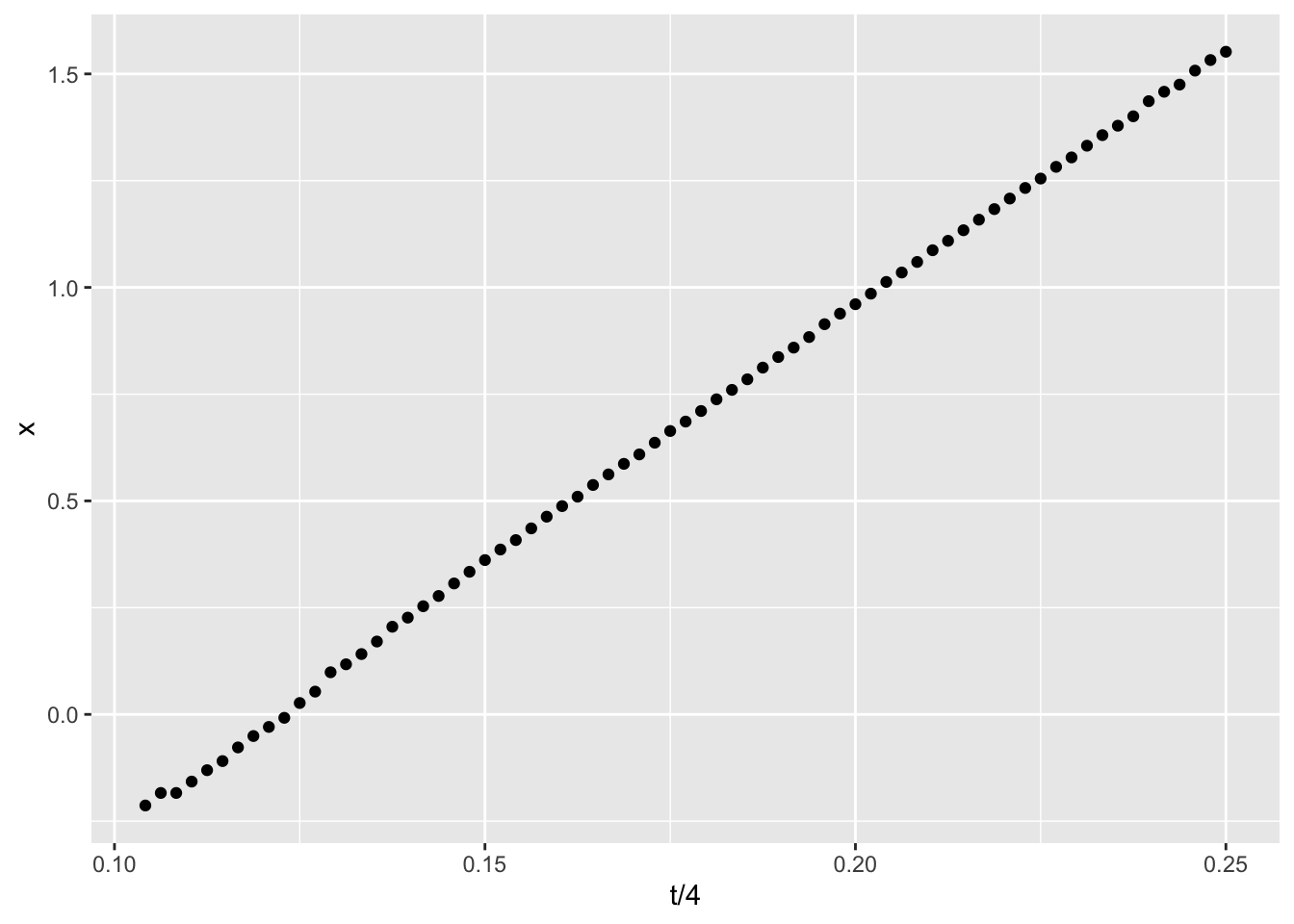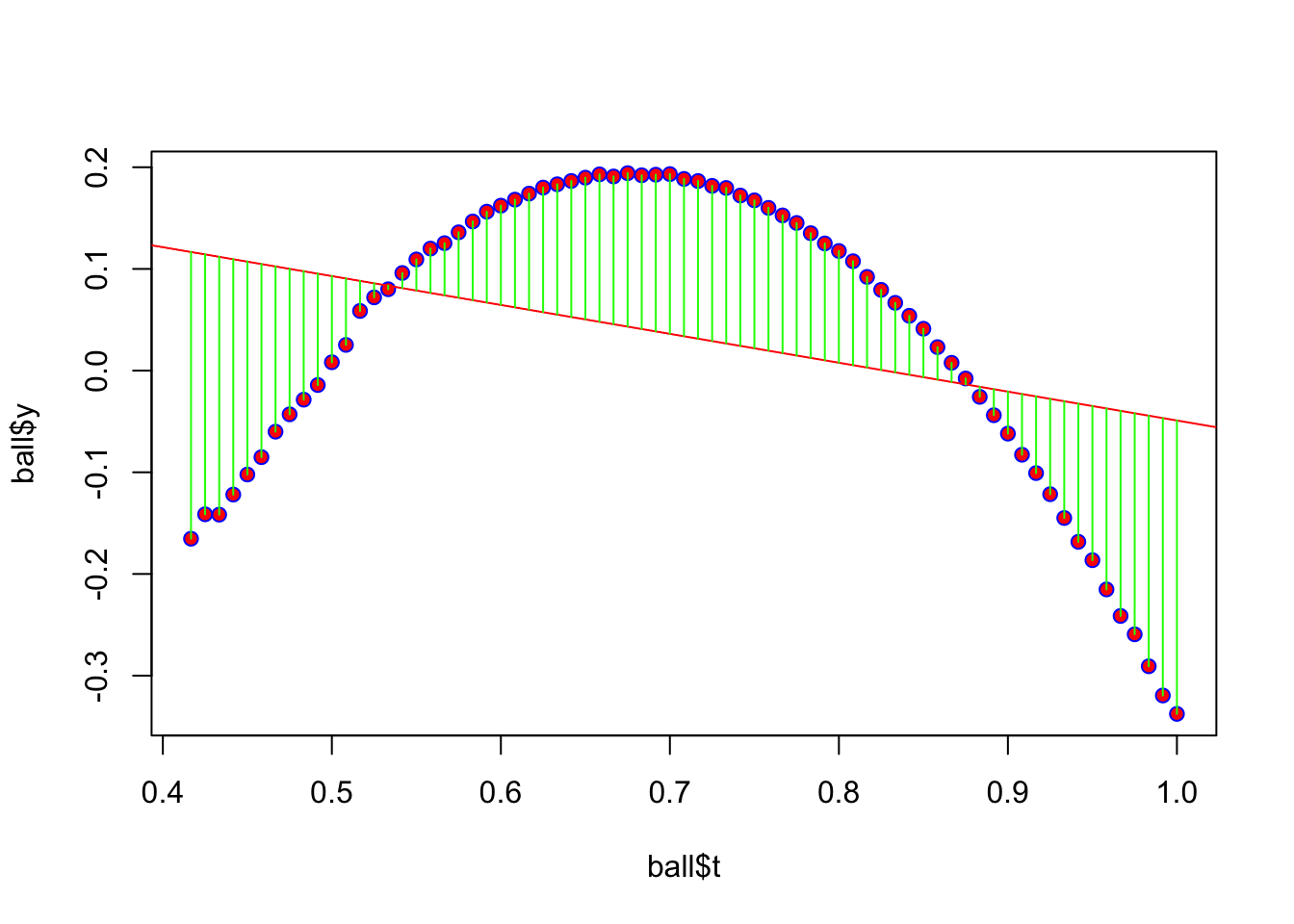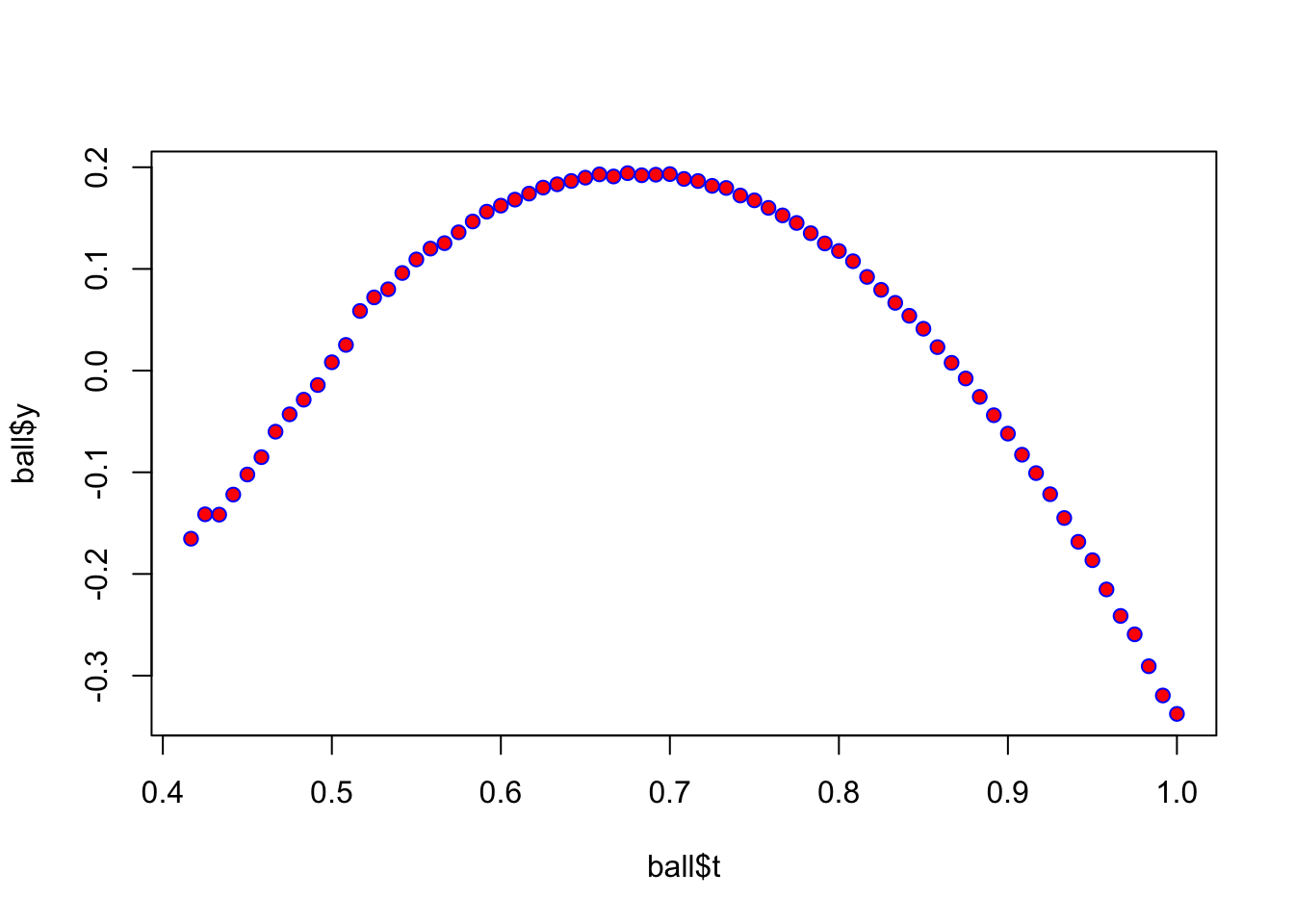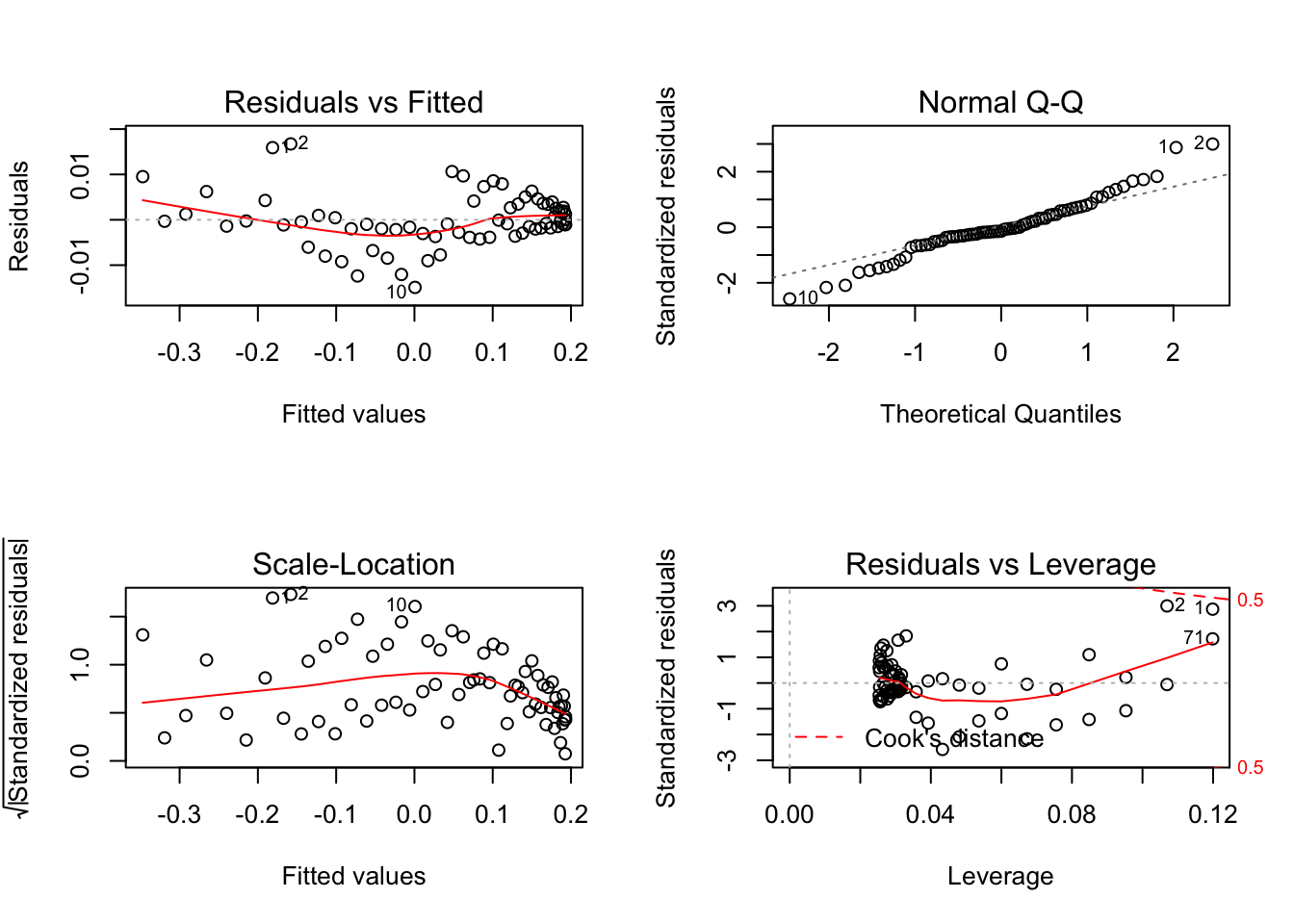# Richard Sprague

My personal website

# Calculating regressions from a physics experiment

Created: 2017-10-08 ; Updated: 2017-10-08

Think of this as a test post, as I try to get this site up and running (in R blogdown), and also while trying to solve a problem set posted by our high school physics AP class.

The raw data comes from a Video Physics, an iPhone/iPad app that captures x-y position data frame-by-frame from a video, in this case a ball that was thrown across the room. Once captured, the data was saved to a CSV file that I read into the variable ball and clean up as follows:

names(ball) <- c("t4","x","y","xv","yv")
ball$t <- ball$t4/4
head(ball)
##         t4         x         y       xv       yv         t
## 1 1.666667 -0.213333 -0.165333 0.679998 0.550665 0.4166667
## 2 1.700000 -0.183999 -0.141333 0.509998 0.401082 0.4250000
## 3 1.733333 -0.183999 -0.141666 0.510665 0.383532 0.4333333
## 4 1.766667 -0.157333 -0.121933 0.699998 0.524220 0.4416667
## 5 1.800000 -0.130666 -0.102200 0.737775 0.561942 0.4500000
## 6 1.833333 -0.109333 -0.085133 0.795553 0.607553 0.4583333
library(tidyr)
#ball.tidy <- gather(ball,x:y,coord,-t,-xv,-yv)
names(ball)
##  "t4" "x"  "y"  "xv" "yv" "t"

The data was captured in slow motion, only 4 frames per second, so to convert everything to seconds, I had to divide the time t by 4.

In throwing the ball across the room, we expect the horizontal component of the motion vectors to move in a straight line. Sure enough, that’s what we see when plotting the x (horizontal) motion against time:

library(ggplot2)

ggplot(data=ball, aes(x=t/4,y=x)) + geom_point()Now use the R lm() function to build a linear model of the motion for that ball. Because I’m using a simple non-quadratic equation, a regression (computed with predict()) shows a linear trend line full of errors. Here is a plot showing the errors (“residuals”) marked in green. If you add up all the green lines (positive and negative), you’d find they sum to zero.

plot(ball$t,ball$y,pch=21,col="blue",bg="red")
model1 <- lm(ball$y~ball$t)
abline(model1,col="red")
yhat <- predict(model1,actual=y)
join <- function(i)
lines(c(ball$t[i],ball$t[i]),c(ball$y[i],yhat[i]),col="green") ignore = sapply(1:nrow(ball),join)Let’s try a non-linear, quadratic version using the same data plot(ball$t,ball$y,pch=21,col="blue",bg="red")model2 <- lm(ball$y~ball$t+I(ball$t^2))
#windows(7,7)
par(mfrow=c(2,2))
plot(model2)#
# abline(model2,col="yellow")
# yhat <- predict(model2,actual=y)
# join <- function(i)
#  lines(c(ball$t[i],ball$t[i]),c(ball$y[i],yhat[i]),col="green") #sapply(1:nrow(ball),join) Study the difference between these two models (linear vs quadratic): model2 ## ## Call: ## lm(formula = ball$y ~ ball$t + I(ball$t^2))
##
## Coefficients:
## (Intercept)       ball$t I(ball$t^2)
##      -2.284        7.269       -5.332
anova(model1,model2)
## Analysis of Variance Table
##
## Model 1: ball$y ~ ball$t
## Model 2: ball$y ~ ball$t + I(ball\$t^2)
##   Res.Df     RSS Df Sum of Sq     F    Pr(>F)
## 1     69 1.37519
## 2     68 0.00237  1    1.3728 39315 < 2.2e-16 ***
## ---
## Signif. codes:  0 '***' 0.001 '**' 0.01 '*' 0.05 '.' 0.1 ' ' 1

Model 2 (the quadratic equation) is clearly superior, showing an extremely low P value for the probability that this data would map so closely to our model based on chance alone.